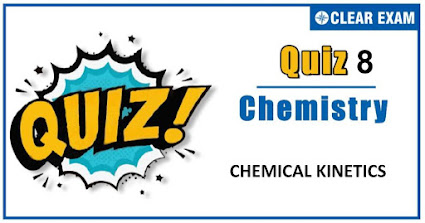## [LATEST]\$type=sticky\$show=home\$rm=0\$va=0\$count=4\$va=0

Q2.Following mechanism has been proposed for a reaction, 2A+B⟶D+E A+B⟶C+D …(Slow) A+C⟶E …(fast) The rate law expression for the reaction is:
•  r=K[A]^2 [B]
•  r=K[A][B]
•  r=K[A]^2
•  r=K[A][C]
Solution
The rate expression is derived for slowest step of mechanism.

Q3.  The differential rate expression for the reaction H_2+I_2⟶2HI is:
•   (-d[H_2])/dt=(-d[I_2])/dt=(-d[HI])/dt
•  (d[H_2])/dt=(d[I_2])/dt=(d[HI])/dt
•  1/2 (d[H_2])/dt=1/2 (d[I_2])/dt=(d[HI])/dt
•  -2 d[H_2 ]/dt=-2 d[I_2 ]/dt=(d[HI])/dt
Solution
Follow review of rate of reaction
Q5.For the reaction N_2+3H_2⟶2NH_3, the rate (d[NH_3])/dt=2×〖10〗^(-4) M s^(-1). Therefore, the rate -(d[N_2])/dt is given as:
•  〖10〗^(-4) M s^(-1)
•  〖10〗^4 M s^(-1)
•  〖10〗^(-2) M s^(-1)
•  〖10〗^(-4) s M^(-1)
Solution
(-d[N_2])/dt=1/2(d[NH_3])/dt

Q6. The rate of chemical reaction (except zero order):
•  Remains constant throughout
•  Decreases from moment to moment
• Is independent of the order of reaction
•  None of the above
Solution
Rate is dx/dt or-d[A]/dt; Where x stands for product concentration and [A] stands for reactant concentration. It continuously decreases with decrease in concentration of reactant with time.

Q7.In a reaction 2A⟶ Products; the concentration of A decreases from 0.5 mol litre^(-1) to 0.4 mol litre^(-1) in 10 minute. The rate of reaction during this interval is:
•  0.05 M min^(-1)
•  5 M min^(-1)
•  0.005 M min^(-1)
•  0.5 M min^(-1)
Solution
Rate of reaction =1/2 [(-d[A])/dt]=1/2 [(0.5-0.4)/10] =0.005 mol litre^(-1) min^(-1).

Q8.The rate law for the chemical reaction 2NO_2CL→2NO_2+CL_2is rate =k[NO_2Cl].The rate determining step is
•  NO_2+Cl_2→NO_2 Cl+Cl
•  NO_2 Cl→NO_2+Cl
•  2NO_2 Cl→2NO_2+2Cl
•  NO_2 Cl+Cl→NO_2+Cl_2
Solution
Rate =k[〖NO〗_2 Cl] Hence ,rate determining step is 〖NO〗_2 CL→〖NO〗_2+CL

Q9.The rate of the reaction A→ product, at the initial concentration of 3.24×〖10〗^(-2) M is nine times its rate at another initial concentration of 1.2×〖10〗^(-3) M. The order of the reaction is
•  1/2
•  3/4
•  3/2
•  2/3
Solution
9=((3.24×〖10〗^(-2))/(1.2×〖10〗^(-3) ))^n 9=(3^3 )^(2/3) order of the reaction is=2/3

Q10. K for a zero order reaction is 2×〖10〗^(-2) mol L^(-1) sec^(-1). If the concentration of the reactant after 25 sec is 0.5 M, the initial concentration must have been:
•  1.25 M
•  1.0 M
•  12.5 M
• 0.5 M
Solution
For zero order [A]_t=[A]_0-kt 0.5=[A]_0-2×〖10〗^(-2)×25 ∴ [A]_0=1.0 M#### Written by: AUTHORNAME

AUTHORDESCRIPTION## Want to know more

Please fill in the details below:

## Latest NEET Articles\$type=three\$c=3\$author=hide\$comment=hide\$rm=hide\$date=hide\$snippet=hide

Name

ltr
item
BEST NEET COACHING CENTER | BEST IIT JEE COACHING INSTITUTE | BEST NEET, IIT JEE COACHING INSTITUTE: CHEMICAL KINETICS QUIZ-8
CHEMICAL KINETICS QUIZ-8